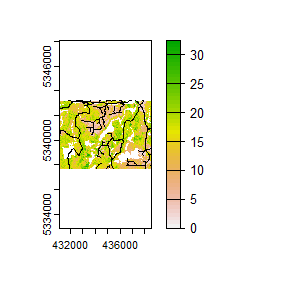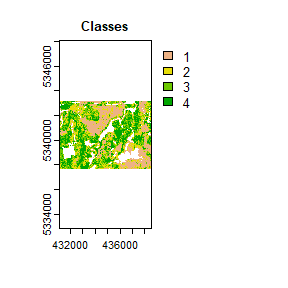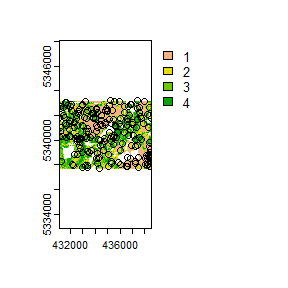# sgsR

## Algorithm structure

sgsR is primarily scripted using the tidyverse, terra package to handle raster processing, and sf package for vector manipulation. Currently, there are 4 primary function verbs that this package uses:

• strat_* - Stratify verb directs the functions to apply stratification algorithms to the input metrics raster mraster and produce stratified raster sraster as the output.

• sample_* - Sample verb directs the functions to extract samples from srasters, which is produced from strat_* functions. Few algorithms (such as sample_srs(), sample_balanced(), sample_systematic()) are capable of using mrasters as the input because those algorithms do not depend on stratified inputs for sampling.

• calculate_* - Calculate verb directs the functions to perform calculations; values derived from these calculations are used in subsequent processing. Predefined sample analysis algorithms (such as calculate_representation(), calculate_coobs()) are included.

• extract_* - Derive raster data for each co-located sample. Includes extract_metrics() for deriving mraster data, and extract_strata() for deriving stratum from srasters. Both functions are used internally within sgsR.

## Example data

We demonstrate and provide examples for functions using sgsR internal data. Use the following code to load data for mraster and road access. Follow along on your own device to explore different outputs and better comprehend the package functions.

### Metrics rasters - mraster

library(sgsR)
library(terra)
library(sf)

#--- Load mraster from internal data ---#
r <- system.file("extdata", "mraster.tif", package = "sgsR")

#--- load mraster using the terra package ---#
mraster <- terra::rast(r)

### access data

a <- system.file("extdata", "access.shp", package = "sgsR")

#--- load the access vector using the sf package ---#
#> Reading layer access' from data source
#>   C:\Users\goodb\AppData\Local\Temp\RtmpshiRyG\Rinst218c452d5988\sgsR\extdata\access.shp'
#>   using driver ESRI Shapefile'
#> Simple feature collection with 167 features and 2 fields
#> Geometry type: MULTILINESTRING
#> Dimension:     XY
#> Bounding box:  xmin: 431100 ymin: 5337700 xmax: 438560 ymax: 5343240
#> Projected CRS: UTM_Zone_17_Northern_Hemisphere
terra::plot(mraster$zq90) terra::plot(access, add = TRUE, col = "black")From the plot output we see the first band (zq90) of the mraster with the access vector overlaid. ## Stratified rasters - sraster In this tutorial, I am going to demonstrate how to produce basic sraster and existing sample data, which will be used in subsequent examples. To produce sraster, we use strat_quantiles(). This function used the input mraster$zq90 distribution and divides it into 4 equally sized quantiles.

#--- apply kmeans algorithm to metrics raster ---#
sraster <- strat_quantiles(mraster = mraster$zq90, # use mraster as input for sampling nStrata = 4, # algorithm will produce 4 strata plot = TRUE) # algorithm will plot outputAfter sraster is produced, use sample_strat() to perform stratified sampling within our sraster to generate a representative sample output based on strata. #--- apply stratified sampling ---# existing <- sample_strat(sraster = sraster, # use mraster as input for sampling nSamp = 200, # request 200 samples be taken mindist = 100, # define that samples must be 100 m apart plot = TRUE) # algorithm will plot output## %>% The sgsR package leverages the %>% operator from the magrittr package. This allows us to “pipe” operations together to save in the amount of code needed to achieve an outcome. A simple example is demonstrated below. #--- non piped ---# sraster <- strat_quantiles(mraster = mraster$zq90, # use mraster as input for sampling
nStrata = 4) # algorithm will produce 4 strata

existing <- sample_strat(sraster = sraster, # use mraster as input for sampling
nSamp = 200, # request 200 samples be taken
mindist = 100) # define that samples must be 100 m apart

extract_metrics(mraster = mraster,
existing = existing)

#--- piped ---#
strat_quantiles(mraster = mraster\$zq90, nStrata = 4) %>%
sample_strat(., nSamp = 200, mindist = 100) %>%
extract_metrics(mraster = mraster, existing = .)`